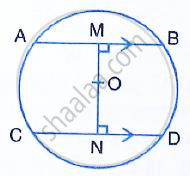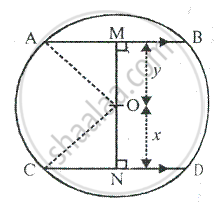Share

In the Figure, Given Below, Ab and Cd Are Two Parallel Chords and O is the Centre. If the Radius of the Circle is 15 Cm, Fins the Distance Mn Between the Two Chords of Lengths 24 Cm and 18 Cm Respecti - ICSE Class 10 - Mathematics

ConceptArc and Chord Properties - Angle in a Semi-circle is a Right Angle

Question

In the figure, given below, AB and CD are two parallel chords and O is the centre. If the radius of the circle is 15 cm, fins the distance MN between the two chords of lengths 24 cm and 18 cm respectively.SolutionGiven – AB = 24 cm, cd = 18 cm
⇒ AM = 12 cm, CN = 9 cm Also, OA = OC = 15 cm
Let MO = y cm, and ON = x cm In right angled ∆AMO
(OA)^2 = (AM)^2 + (OM)^2

⇒ 15^2 = 12^2 + y^2

⇒ y^2 = 15^2 -12^2

⇒ y^2 = 225 -144

⇒ y^2 = 81
⇒ y = 9 cm
In right angled ΔCON
(OC)^2 = (ON)^2 + (CN)^2

⇒ 15^2 = x^2 + 9^2
⇒ x^2 =15^2 - 9^2
⇒ x^2 = 225 - 81

⇒ x^2 = 144
⇒ y = 12 cm
Now, MN = MO + ON
= y + x
= 9 cm +12 cm
= 21 cm

Is there an error in this question or solution?

Video TutorialsVIEW ALL 

Solution In the Figure, Given Below, Ab and Cd Are Two Parallel Chords and O is the Centre. If the Radius of the Circle is 15 Cm, Fins the Distance Mn Between the Two Chords of Lengths 24 Cm and 18 Cm Respecti Concept: Arc and Chord Properties - Angle in a Semi-circle is a Right Angle.
S